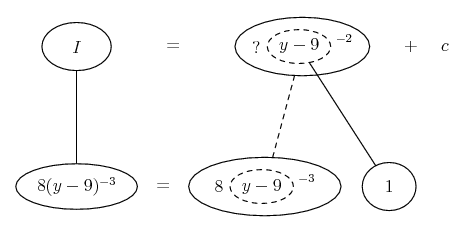# Thread: Finding the integral, can i get my answer checked?

1. ## Finding the integral, can i get my answer checked?

$\displaystyle \int\frac{8}{(y-9)^3}$dy

2. I get an answer of $\displaystyle \frac{4}{(y-9)^2}+c$ is this correct?

3. It is the same as $\displaystyle \int {8u^{ - 3} du}$.

4.Originally Posted by PlatoIt is the same as $\displaystyle \int {8u^{ - 3} du}$.
Yes if you let U=y-9.

5. So is my answer right then?

6.Originally Posted by Darkhrse99So is my answer right then?
Yes. But I must ask why you don't know that it is correct?

7.Originally Posted by PlatoYes. But I must ask why you don't know that it is correct?
that's twice ...

8.Originally Posted by PlatoYes. But I must ask why you don't know that it is correct?
The first time I did it, I was coming up with a negative 4, then I did it again and came with a positive 4. I was just making sure the negative answer I came up with was wrong!

9. Just in case a picture helps ...(and helps with the checking by differentiating, too... check again!)

As usual, straight continuous lines differentiate downwards with respect to y, and the straight dashed line with respect to the dashed balloon expression - so that the triangular network is the chain rule.

Don't integrate - balloontegrate!

http://www.ballooncalculus.org/forum.top.php

#### Search Tags

answer, checked, finding, integral